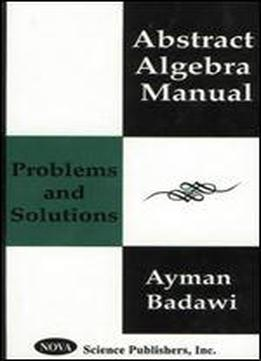# Abstract Algebra Manual: Problems And Solutions by Ayman Badawi / 2001 / English / PDF

This is the most current textbook in teaching the basic concepts of abstract algebra. The author finds that there are many students who just memorise a theorem without having the ability to apply the theorem to a given problem. Therefore, this is a hands-on manual, where many typical algebraic problems are provided for students to be able to apply the theorems and to actually practice the methods they have learned. Each chapter begins with a statement of a major result in Group and Ring Theory, followed by problems and solutions.

views: 345